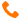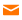Group Services: Technology Consulting+91-9999-283-283/9540-283-283info@sisoft.in
Sisoft

Course outline for Data Analytics(DA)/Machine Learning(ML) With Python

Goal:

The goal of this course is to examine large amounts of data to uncover hidden patterns, correlations and other insights. This help in preparing machine learning model using AI fundamentals

Audience:

This course is designed for any one willing to make career in Data Analytics and Machine Learning .

Pre-requisites:

Any Graduate or Post-Graduate having affinity with Data, Information, Knowledge and Wisdom

Basics of Python

Duration:

60 hours

Course Structure

1. Python Data Science Overview

• What is Data Science
• What Is Data Science and Machine Learning?
• Introduction of Python Data Science Tools
• Setting up environment for this course
• Python Data Science Packages to be used

2. Fundamental of Statistics

• Overview
• Basic Terminology of Statistics
• Variables
• Data
• Statistics
• Dispersion
• Scattering
• Observation
• Time Series Data
• Population
• Sample
• Variation
• Shape
• Data Collections
• Descriptive Statistics
• Data Distribution
• Confidence Interval

3. Statistical Inferences and Relationship Between Variables

• Hypothesis Testing
• Correlation Theory
• Linear regression theory
• Polynomial Regression
• Logistic Regression

4. Introduction to NumPy (Numerical Python)

• Numpy: Introduction
• Create Numpy Arrays
• Numpy Operations
• Matrix Airthmetic and Linear Systems
• Numpy for Basic Matrix Airthmetic
• Solve Equation with Numpy
• Statistical Operations

5. Pandas

• Introduction to Data Structures
• Series
• DataFrame
• Panel
• CSV Data
• Excel Data
• JSON Data
• HTML Data
• Data pre-Processing/Wrangling/Cleaning
• Removing NAs /No Values from data
• Basic Data Handling: Starting with conditional data section
• Drop Column/Rows
• Subset and Index Data
• Basic Data Grouping Based on Qualitative Attributes
• Cross tabulation
• Reshaping
• Pivoting
• Rank and sort Data
• Concatenate
• Merging and Joining frames

4. Data Visualization (plotLib)

• Introduction
• Histograms
• Box Plots
• Scatter Plots
• Bar Plot
• Pie Chart
• Line Chart

7. Machine Learning: Overview

• Machine Learning Languages, Types, and Examples
• Machine Learning vs Statistical Modelling
• Supervised vs Unsupervised Learning
• Python Libraries : skLearn, TensorFlow

8. Supervised Learnings

• K Nearest neighbours
• Decision Trees
• Using Logistic Regression as Classification Model
• RF-Classification
• SVM Linear Classification
• Knn Regression

9. Unsupervised Learning

• Measuring the Distances Between Clusters - Single Linkage Clustering
• Measuring the Distances Between Clusters - Algorithms for Hierarchy Clustering
• Density-Based Clustering

10. Time Series Forecasting

• Time series
• Estimating and Eliminating the Deterministic Components if they are present in the Model.
• Estimating and Eliminating Seasonality if it is present in the Model
• Modeling the Remainder using Auto Regressive Moving Average (ARMA) Models
• Identify ‘order’ of the ARMA model
• ‘Forecast’ or Predict for Future Values

11. Support Vector Machine(SVM)

• Linear Classifiers
• Margin of SVM's
• SVM optimization
• SVM for Data which is not linear separable
• Learning non-linear patterns
• Kernel Trick
• SVM Parameter Tuning
• Linear SVM using Python